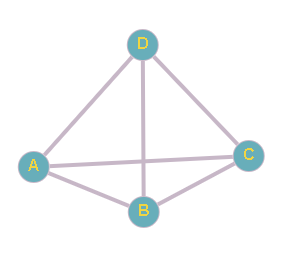# Tetrahedron

Posted: 6 Dec, 2020
Difficulty: Moderate

## PROBLEM STATEMENT

#### For example:``````For n = 2, there are three cycle D -> A -> D, D -> B -> D and D -> C -> D
``````
##### Input Format:
``````The first line of input contains an integer ‘T’, denoting the number of test cases. Then each test case follows.

The first line and the last line of each test case contain the integer ‘N’ denoting the required length of the cyclic path.
``````
##### Output Format:
``````For each test case, print the count the number of ways in which the ant can go from the initial vertex ‘D’ to itself in exactly ‘n’ steps modulo 1000000007 (10^9 + 7).

The output of each test case will be printed on a separate line.
``````
##### Note:
``````You do not need to print anything, it has already been taken care of. Just implement the given function.
``````
##### Constraints:
``````1 <= T <= 5
1 <= N <= 10 ^ 5

Time Limit: 1 sec.
``````Approach 1

Let’s call vertex ‘A’ as 3, ‘B’ as 2, ‘C’ as 1 and ‘D’ as 0.

The idea is to break the main problem into smaller subproblems and recursively solve the smaller subproblems.

Let P(x, n),  represents the number of ways to move from vertex 0 to vertex x in ‘n’ steps.

Then our main problem is P(0, n).

P(0, n ) = P(1, n - 1) + P(2,n -1) + P( 3, n - 2).

Since vertex 1, 2 and 3 are equivalent to each other so we can write, P(0, n) = 3 * P(1, n -1)

And for x != 0 let's say 1.

P(1, n) = 2 * P(1, n - 1) + P(0, n - 1).

Hence we got the optimal substructure property.

## Algorithm :

The steps are as follows:

Let 'numberOfWays' be the function that count all the possible ways

• Take a variable  ‘mod’ value equal to 10 ^ 9 +7.
• Make the helper function ‘count(vertex, steps)’ and call the helper function ‘count(vertex, steps)’ and store the answer in variable  ‘ans’.
• Return ‘ans’ as final result.

Description of ‘count(vertex, steps)’ function

This function returns the number of ways to reach vertex ‘0’ from vertex ‘vertex’  in ‘steps’.

• Check the base condition i.e steps is equal to 0.
• If ‘vertex’ is equal to 0, return 1 way.
• Else return 0.
• Take a variable 'ans', initialize it to 0.
• If it is 0th vertex:
• Store the three times of answer of subproblem(1, steps - 1) in 'ans', and take modulo ‘mod’.
• Else:
• ‘ans’ is equal to 2 times of count(1, steps - 1)  + count(0, steps - 1).
• Update the 'dp[vertex][steps]’ with ‘ans’ i.e dp[vertex][steps] = ans.
• Return 'ans' .